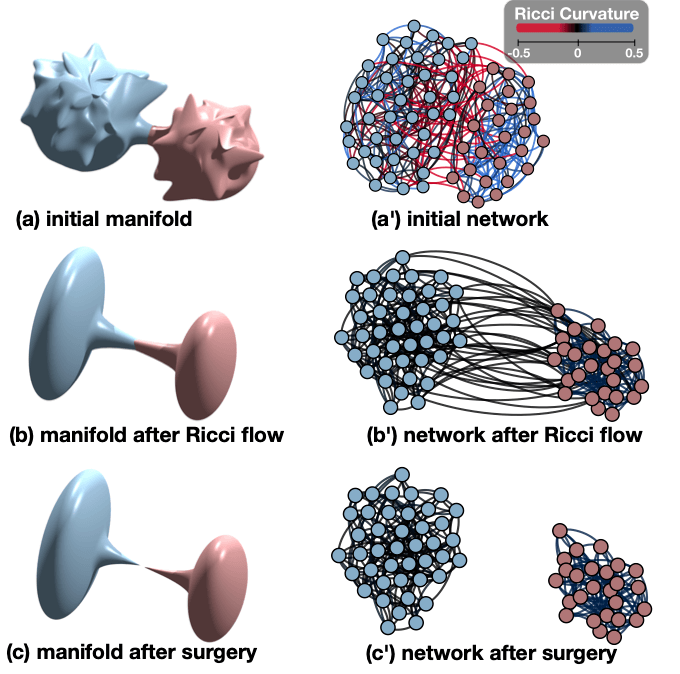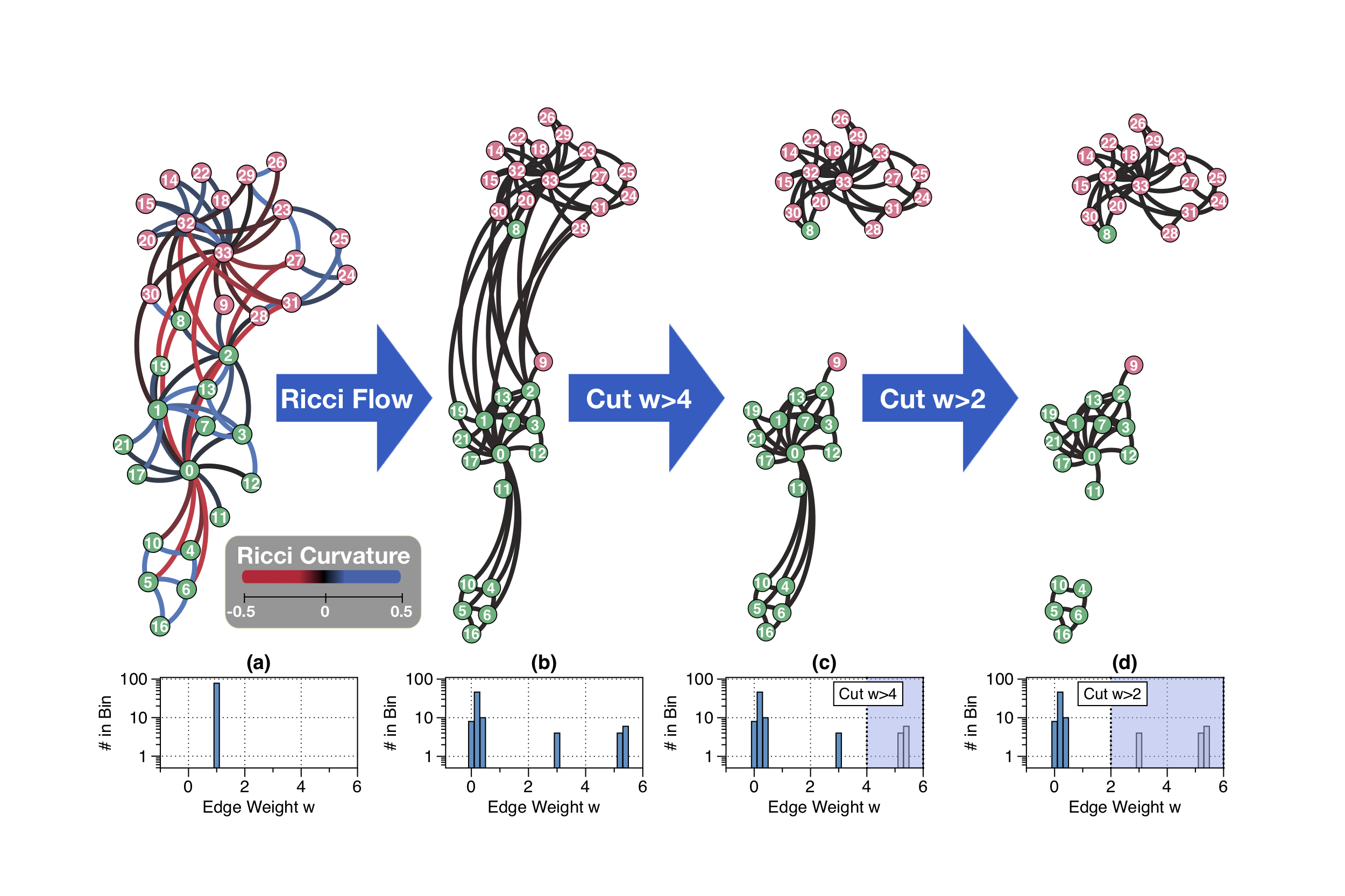# GraphRicciCurvature¶

This work computes the Ollivier-Ricci Curvature , Ollivier-Ricci Flow   and Forman-Ricci Curvature (or Forman curvature) , and Ricci community  detected by Ollivier-Ricci flow metric.Curvature is a geometric property to describe the local shape of an object. If we draw two parallel paths on a surface with positive curvature like a sphere, these two paths move closer to each other while for a negatively curved surface like a saddle, these two paths tend to be apart.

In [Ni], we observe that the edge Ricci curvature plays an important role in the graph structure. An edge with positive curvature represents an edge within a cluster, while a negatively curved edge tent to be a bridge within clusters. Also, negatively curved edges are highly related to graph connectivity, with negatively curved edges removed from a connected graph, the graph soon become disconnected.

Ricci flow is a process to uniformized the edge Ricci curvature of the graph. For a given graph, the Ricci flow gives a “Ricci flow metric” on each edge as edge weights, such that under these edge weights, the Ricci curvature of the graph is mostly equal everywhere. In [Ni3], this “Ricci flow metric” is shown to be able to detect communities.

Both Ricci curvature and Ricci flow metric can act as a graph fingerprint for graph classification. The different graph gives different edge Ricci curvature distributions and different Ricci flow metric.

Video demonstration of Ricci flow for community detection:## Package Requirement¶

• NetworkX (Based Graph library)
• NetworKit (Shortest path algorithm)
• CVXPY (LP solver for Optimal transportation)
• NumPy (CVXPY support)
• POT (For approximate Optimal transportation distance)
• python-louvain (For faster modularity computation)

## Installation¶

### Installing via pip¶

pip3 install [--user] GraphRicciCurvature

• From version 0.4.0, in order to support larger graph, we switch to NetworKit’s pairwise bidirectional dijkstra algorithm for density distribution (NetworKit>6.0 is required). If the installation of NetworKit failed, please refer to [NetworKit’ Installation instructions]. In most of the cast build this package from source is recommended.

To run with the latest code for the best performance, upgrade GraphRicciCurvature to the latest version with pip:

pip3 install [--user] --upgrade GraphRicciCurvature


## Getting Started¶

• See the jupyter notebook tutorial on [nbviewer] for a walk through for the basic usage of Ricci curvature, Ricci flow, and Ricci flow for community detection.
• Or you can run it in directly on [binder] (no account required) or [Google colab] (Faster but Google account required).
• Check the Documentations.
• Try out sample graphs with precomputed Ricci curvature/flow.

### Simple Example¶

import networkx as nx
from GraphRicciCurvature.OllivierRicci import OllivierRicci
from GraphRicciCurvature.FormanRicci import FormanRicci

print("\n- Import an example NetworkX karate club graph")
G = nx.karate_club_graph()

print("\n===== Compute the Ollivier-Ricci curvature of the given graph G =====")
# compute the Ollivier-Ricci curvature of the given graph G
orc = OllivierRicci(G, alpha=0.5, verbose="INFO")
orc.compute_ricci_curvature()
print("Karate Club Graph: The Ollivier-Ricci curvature of edge (0,1) is %f" % orc.G["ricciCurvature"])

print("\n===== Compute the Forman-Ricci curvature of the given graph G =====")
frc = FormanRicci(G)
frc.compute_ricci_curvature()
print("Karate Club Graph: The Forman-Ricci curvature of edge (0,1) is %f" % frc.G["formanCurvature"])

# -----------------------------------
print("\n=====  Compute Ricci flow metric - Optimal Transportation Distance =====")
G = nx.karate_club_graph()
orc_OTD = OllivierRicci(G, alpha=0.5, method="OTD", verbose="INFO")
orc_OTD.compute_ricci_flow(iterations=10)
print("\n=====  Compute Ricci community - by Ricci flow =====")
clustering = orc_OTD.ricci_community()


More example in example.py.

## Reference¶

  Ni, C.-C., Lin, Y.-Y., Gao, J., Gu, X., and Saucan, E. 2015. Ricci curvature of the Internet topology (Vol. 26, pp. 2758–2766). Presented at the 2015 IEEE Conference on Computer Communications (INFOCOM), IEEE. [arXiv]
  Ni, C.-C., Lin, Y.-Y., Gao, J., and Gu, X. 2018. Network Alignment by Discrete Ollivier-Ricci Flow, Graph Drawing 2018, [arXiv]
  (1, 2) Ni, C.-C., Lin, Y.-Y., Luo, F. and Gao, J. 2019. Community Detection on Networks with Ricci Flow, Scientific Reports, [arXiv]
  Sreejith, R. P., Karthikeyan Mohanraj, Jürgen Jost, Emil Saucan, and Areejit Samal. 2016. Forman Curvature for Complex Networks. Journal of Statistical Mechanics: Theory and Experiment 2016 (6). IOP Publishing: 063206. [arXiv]

## Cite¶

If you use this code in your research, please considering cite our paper:

@article{ni2019community,
title={Community detection on networks with ricci flow},
author={Ni, Chien-Chun and Lin, Yu-Yao and Luo, Feng and Gao, Jie},
journal={Scientific reports},
volume={9},
number={1},
pages={1--12},
year={2019},
publisher={Nature Publishing Group}
}